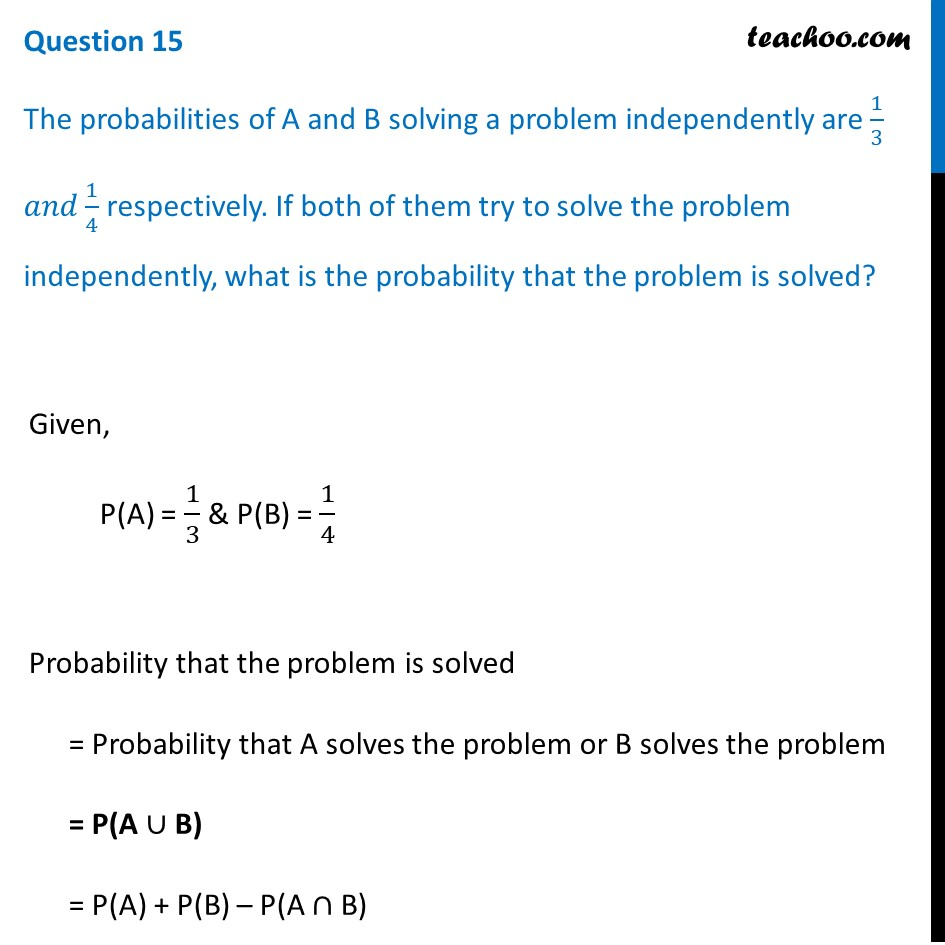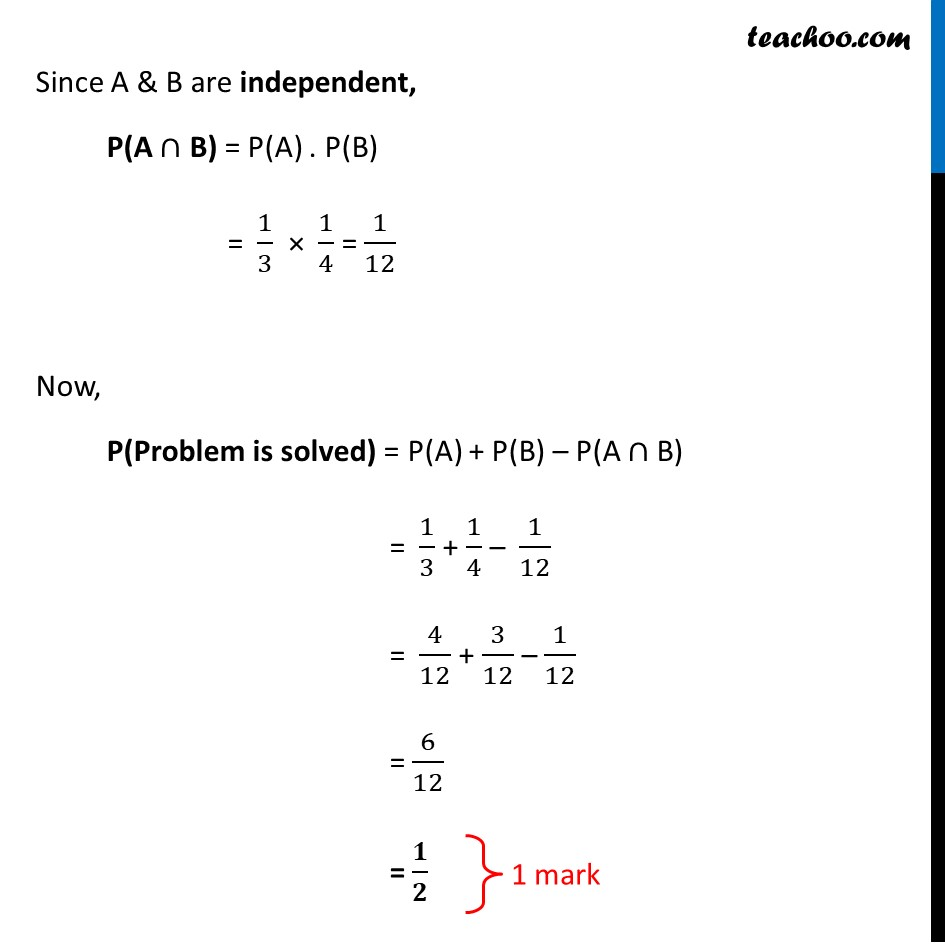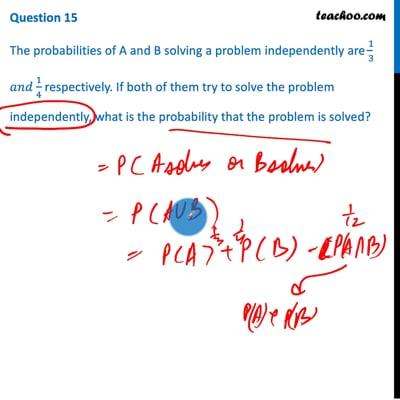CBSE Class 12 Sample Paper for 2021 Boards

Class 12
Solutions of Sample Papers and Past Year Papers - for Class 12 Boards

## The probabilities of A and B solving a problem independently are 1/3 𝑎𝑛𝑑 1/4 respectively. If both of them try to solve the problem independently, what is the probability that the problem is solved?This video is only available for Teachoo black users

Note : This is similar to Ex 13.2, 14 of NCERT – Chapter 13 Class 12 Probability

Introducing your new favourite teacher - Teachoo Black, at only ₹83 per month

### Transcript

Question 15 The probabilities of A and B solving a problem independently are 1/3 𝑎𝑛𝑑 1/4 respectively. If both of them try to solve the problem independently, what is the probability that the problem is solved? Given, P(A) = 1/3 & P(B) = 1/4 Probability that the problem is solved = Probability that A solves the problem or B solves the problem = P(A ∪ B) = P(A) + P(B) – P(A ∩ B) Since A & B are independent, P(A ∩ B) = P(A) . P(B) = 1/3 × 1/4 = 1/12 Now, P(Problem is solved) = P(A) + P(B) – P(A ∩ B) = 1/3 + 1/4 – 1/12 = 4/12 + 3/12 – 1/12 = 6/12 = 𝟏/𝟐